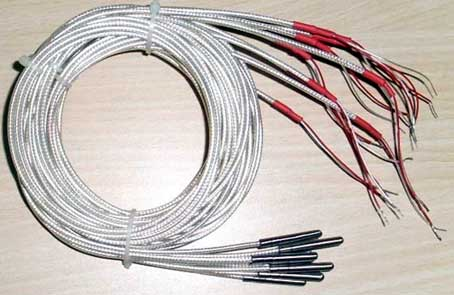﻿ What does pt100 mean?_

China sensor manufacturers

# What does pt100 mean?

The PT100 is a temperature sensor and is a platinum wire thermal resistance sensor with good stability and linearity. Can work in the range of -200 ° C to 650 ° C.

A Resistive Temperature Detector (RTD) is a resistor made of a material. It changes the resistance value as the temperature rises. If it rises with the temperature and the resistance value rises, it is called the positive resistivity. If it rises with temperature and the resistance value decreases, it is called negative resistance coefficient. Most resistive temperature detectors are made of metal. Among them, the resistive temperature detector made of platinum (Pt) is the most stable - acid and alkali resistant, does not deteriorate, is quite linear... and is most used by industry.

The PT100 temperature sensor is a resistive temperature detector made of platinum (Pt) and is a positive resistivity. The relationship between resistance and temperature change is as follows:
R = Ro (1 + αT) where α = 0.00392 and Ro is 100 Ω (resistance at 0 ° C). T is Celsius temperature. Therefore, the resistive temperature detector made of platinum is also called PT100. 1: Vo = 2.55 mA × 100 (1 + 0.00392 T) = 0.255 + T / 1000.

2, When measuring Vo, no current can be separated, otherwise the measured value will be inaccurate. Circuit Analysis Since the power supply is more noisy after the general power supply, we use the Zener diode as the voltage regulator. Due to the action of the 7.2V Zener diode, the voltage sum of the 1K resistor and the 5K variable resistor is 6.5V. The emitter (collector) pole current of the transistor is determined by the adjustment of the 5K variable resistor. And we have to adjust the collector current to 2.55mA, so that the measurement voltage V is 0.255+T/1000 as indicated by the arrow. Subsequent non-inverting amplifiers, the input resistance is almost infinite, and at the same time amplified 10 times, making the op amp output 2.55 + T / 100. The role of the 6V Zener diode is the effect of the 7.2V Zener diode. We use it to call 2.55V, so the output voltage V1 of the voltage follower is also 2.55V. The output of the differential amplifier is then Vo=10(V2-V1)=10(2.55+T/100-2.55)=T/10. If the room temperature is now 25°C, the output voltage is 2.5V.﻿
﻿